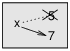# 7.1: Reassignment

$$\newcommand{\vecs}{\overset { \rightharpoonup} {\mathbf{#1}} }$$ $$\newcommand{\vecd}{\overset{-\!-\!\rightharpoonup}{\vphantom{a}\smash {#1}}}$$$$\newcommand{\id}{\mathrm{id}}$$ $$\newcommand{\Span}{\mathrm{span}}$$ $$\newcommand{\kernel}{\mathrm{null}\,}$$ $$\newcommand{\range}{\mathrm{range}\,}$$ $$\newcommand{\RealPart}{\mathrm{Re}}$$ $$\newcommand{\ImaginaryPart}{\mathrm{Im}}$$ $$\newcommand{\Argument}{\mathrm{Arg}}$$ $$\newcommand{\norm}{\| #1 \|}$$ $$\newcommand{\inner}{\langle #1, #2 \rangle}$$ $$\newcommand{\Span}{\mathrm{span}}$$ $$\newcommand{\id}{\mathrm{id}}$$ $$\newcommand{\Span}{\mathrm{span}}$$ $$\newcommand{\kernel}{\mathrm{null}\,}$$ $$\newcommand{\range}{\mathrm{range}\,}$$ $$\newcommand{\RealPart}{\mathrm{Re}}$$ $$\newcommand{\ImaginaryPart}{\mathrm{Im}}$$ $$\newcommand{\Argument}{\mathrm{Arg}}$$ $$\newcommand{\norm}{\| #1 \|}$$ $$\newcommand{\inner}{\langle #1, #2 \rangle}$$ $$\newcommand{\Span}{\mathrm{span}}$$$$\newcommand{\AA}{\unicode[.8,0]{x212B}}$$

As you may have discovered, it is legal to make more than one assignment to the same variable. A new assignment makes an existing variable refer to a new value (and stop referring to the old value).

>>> x = 5
>>> x
5
>>> x = 7
>>> x
7


The first time we display x, its value is 5; the second time, its value is 7.

Figure $$\PageIndex{1}$$ shows what reassignment looks like in a state diagram.

At this point I want to address a common source of confusion. Because Python uses the equal sign (=) for assignment, it is tempting to interpret a statement like a = b as a mathematical proposition of equality; that is, the claim that a and b are equal. But this interpretation is wrong.

First, equality is a symmetric relationship and assignment is not. For example, in mathematics, if a=7 then 7=a. But in Python, the statement a = 7 is legal and 7 = a is not.

Also, in mathematics, a proposition of equality is either true or false for all time. If a=b now, then a will always equal b. In Python, an assignment statement can make two variables equal, but they don’t have to stay that way:

>>> a = 5
>>> b = a    # a and b are now equal
>>> a = 3    # a and b are no longer equal
>>> b
5


The third line changes the value of a but does not change the value of b, so they are no longer equal.

Reassigning variables is often useful, but you should use it with caution. If the values of variables change frequently, it can make the code difficult to read and debug.Figure $$\PageIndex{1}$$: State diagram.

This page titled 7.1: Reassignment is shared under a CC BY-NC 3.0 license and was authored, remixed, and/or curated by Allen B. Downey (Green Tea Press) via source content that was edited to the style and standards of the LibreTexts platform; a detailed edit history is available upon request.